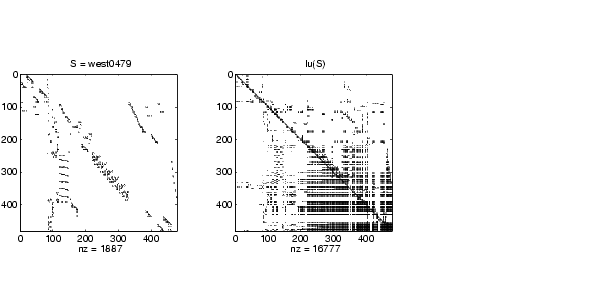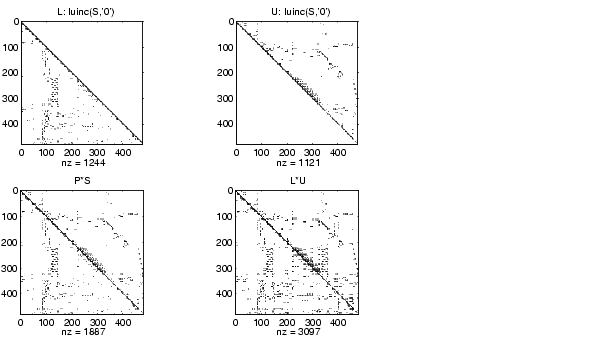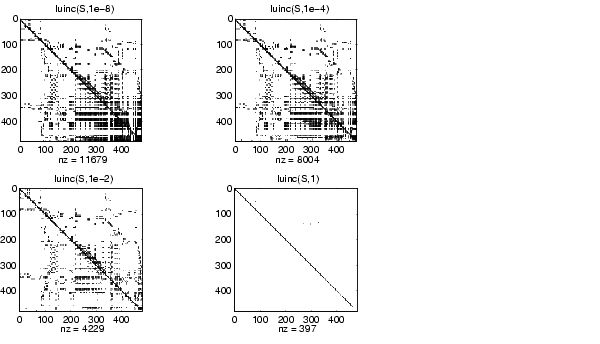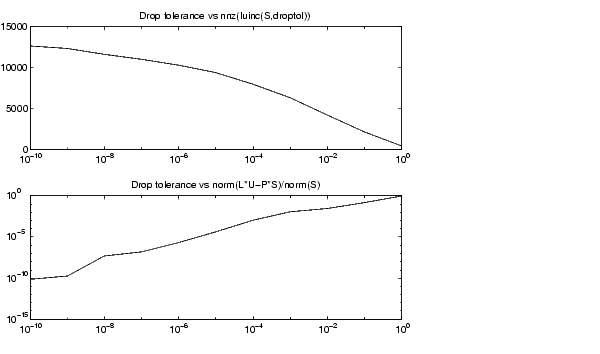MATLAB Function Referenceluinc

Incomplete LU matrix factorizations

Syntax

• ```luinc(X,'0')
[L,U] = luinc(X,'0')
[L,U,P] = luinc(X,'0')
luinc(X,droptol)
luinc(X,options)
[L,U] = luinc(X,options)
[L,U] = luinc(X,droptol)
[L,U,P] = luinc(X,options)
[L,U,P] = luinc(X,droptol)
```

Description

`luinc` produces a unit lower triangular matrix, an upper triangular matrix, and a permutation matrix.

```luinc(X,'0') ``` computes the incomplete LU factorization of level 0 of a square sparse matrix. The triangular factors have the same sparsity pattern as the permutation of the original sparse matrix `X,` and their product agrees with the permuted `X` over its sparsity pattern. `luinc(X,'0')` returns the strict lower triangular part of the factor and the upper triangular factor embedded within the same matrix. The permutation information is lost, but `nnz(luinc(X,'0')) = nnz(X)`, with the possible exception of some zeros due to cancellation.

```[L,U] = luinc(X,'0') ``` returns the product of permutation matrices and a unit lower triangular matrix in `L` and an upper triangular matrix in `U`. The exact sparsity patterns of `L`, `U,` and `X` are not comparable but the number of nonzeros is maintained with the possible exception of some zeros in `L` and `U` due to cancellation:

• ```  nnz(L)+nnz(U) = nnz(X)+n, where `X` is `n`-by-`n`.
```

The product `L*U` agrees with `X` over its sparsity pattern. `(L*U).*spones(X)-X `has entries of the order of `eps`.

```[L,U,P] = luinc(X,'0') ``` returns a unit lower triangular matrix in `L`, an upper triangular matrix in `U` and a permutation matrix in `P`. `L` has the same sparsity pattern as the lower triangle of the permuted `X`

• ```  spones(L) = spones(tril(P*X))
```

with the possible exceptions of `1`s on the diagonal of `L` where `P*X` may be zero, and zeros in `L `due to cancellation where `P*X` may be nonzero. `U` has the same sparsity pattern as the upper triangle of `P*X`

• ```  spones(U) = spones(triu(P*X))
```

with the possible exceptions of zeros in `U` due to cancellation where `P*X` may be nonzero. The product `L*U` agrees within rounding error with the permuted matrix `P*X` over its sparsity pattern. `(L*U).*spones(P*X)-P*X` has entries of the order of `eps`.

```luinc(X,droptol) ``` computes the incomplete LU factorization of any sparse matrix using a drop tolerance. `droptol` must be a non-negative scalar. `luinc(X,droptol)` produces an approximation to the complete LU factors returned by `lu(X)`. For increasingly smaller values of the drop tolerance, this approximation improves, until the drop tolerance is `0`, at which time the complete LU factorization is produced, as in `lu(X)`.

As each column `j` of the triangular incomplete factors is being computed, the entries smaller in magnitude than the local drop tolerance (the product of the drop tolerance and the norm of the corresponding column of `X)`

• ```  droptol*norm(X(:,j))
```

are dropped from the appropriate factor.

The only exceptions to this dropping rule are the diagonal entries of the upper triangular factor, which are preserved to avoid a singular factor.

```luinc(X,options) ``` specifies a structure with up to four fields that may be used in any combination: `droptol`, `milu`, `udiag`, `thresh`. Additional fields of `options` are ignored.

`droptol` is the drop tolerance of the incomplete factorization.

If `milu` is `1`, `luinc` produces the modified incomplete LU factorization that subtracts the dropped elements in any column from the diagonal element of the upper triangular factor. The default value is` 0`.

If `udiag` is `1`, any zeros on the diagonal of the upper triangular factor are replaced by the local drop tolerance. The default is `0`.

`thresh` is the pivot threshold between `0` (forces diagonal pivoting) and `1`, the default, which always chooses the maximum magnitude entry in the column to be the pivot. `thresh` is described in greater detail in `lu`.

`luinc(X,options)` is the same as `luinc(X,droptol)` if options has `droptol` as its only field.

```[L,U] = luinc(X,options) ``` returns a permutation of a unit lower triangular matrix in `L` and an upper triangular matrix in `U`. The product `L*U` is an approximation to `X`. `luinc(X,options)` returns the strict lower triangular part of the factor and the upper triangular factor embedded within the same matrix. The permutation information is lost.

[L,U] = `luinc(X,options)` is the same as `luinc(X,droptol)` if options has `droptol` as its only field.

```[L,U,P] = luinc(X,options) ``` returns a unit lower triangular matrix in `L`, an upper triangular matrix in `U,` and a permutation matrix in `P`. The nonzero entries of `U` satisfy

• ```  abs(U(i,j)) >= droptol*norm((X:,j)),
```

with the possible exception of the diagonal entries which were retained despite not satisfying the criterion. The entries of `L` were tested against the local drop tolerance before being scaled by the pivot, so for nonzeros in `L`

• ```abs(L(i,j)) >= droptol*norm(X(:,j))/U(j,j).
```

The product `L*U` is an approximation to the permuted `P*X`.

`[L,U,P] = luinc(X,options)` is the same as `[L,U,P] = luinc(X,droptol)` if `options` has `droptol` as its only field.

Remarks

These incomplete factorizations may be useful as preconditioners for solving large sparse systems of linear equations. The lower triangular factors all have `1`s along the main diagonal but a single `0` on the diagonal of the upper triangular factor makes it singular. The incomplete factorization with a drop tolerance prints a warning message if the upper triangular factor has zeros on the diagonal. Similarly, using the `udiag` option to replace a zero diagonal only gets rid of the symptoms of the problem but does not solve it. The preconditioner may not be singular, but it probably is not useful and a warning message is printed.

Limitations

luinc(X,'0') works on square matrices only.

Examples

Start with a sparse matrix and compute its LU factorization.

• ```load west0479;
S = west0479;
LU = lu(S);```

Compute the incomplete LU factorization of level 0.

• ```[L,U,P] = luinc(S,'0');
D = (L*U).*spones(P*S)-P*S;
```

`spones(U)` and `spones(triu(P*S))` are identical.

`spones(L)` and `spones(tril(P*S))` disagree at 73 places on the diagonal, where `L` is `1` and` P*S` is `0,` and also at position (206,113), where `L` is `0` due to cancellation, and `P*S` is `-1`. `D` has entries of the order of eps.• ```[IL0,IU0,IP0] = luinc(S,0);
[IL1,IU1,IP1] = luinc(S,1e-10);
.
.
.
```

A drop tolerance of `0` produces the complete LU factorization. Increasing the drop tolerance increases the sparsity of the factors (decreases the number of nonzeros) but also increases the error in the factors, as seen in the plot of drop tolerance versus `norm(L*U-P*S,1)/norm(S,1)` in the second figure below.Algorithm

`luinc(X,'0')` is based on the "KJI" variant of the LU factorization with partial pivoting. Updates are made only to positions which are nonzero in `X`.

`luinc(X,droptol)` and `luinc(X,options`) are based on the column-oriented `lu` for sparse matrices.

See Also

`lu`, `cholinc`, `bicg`

References

  Saad, Yousef, Iterative Methods for Sparse Linear Systems, PWS Publishing Company, 1996, Chapter 10 - Preconditioning Techniques.

© 1994-2005 The MathWorks, Inc.